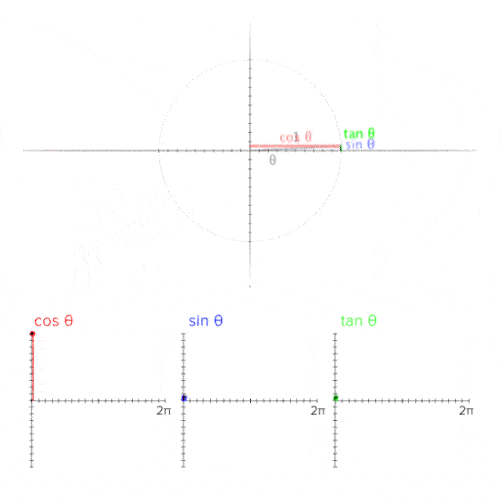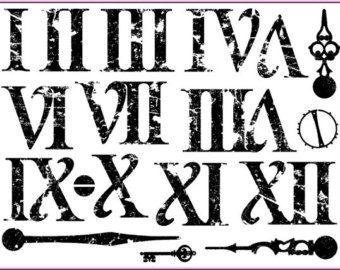# 14 Interesting Facts About Mathematics

Numbers are one of the significant parts of our lives. From studies, career, daily chores to relationships, everything is related to numbers. Therefore Mathematics proves to be an essential subject for students. Even though numbers can be scary sometimes, but if learned properly and with fun, they can be pretty amazing and cool. To make our point, let’s see few interesting facts about mathematics:1. Hundred in reality means 120Confusing as it may sound after the heading, the weirdly interesting thing is that the word “hundred” is derived from another word “hundrath” which actually means 120. Not very logical thing for this logic subject!
Source: toplearning.com

2. The Popular number of allWell if math would be a high school, number 7 would be the most popular number of all. It is because of many reasons like this is “arithmetically unique”. It is the only number you can’t really multiply or divide and still keep it in that group.
Source: toplearning.comThe lifeline of today’s time, Google search engine is a term which is derived from word “googol” which is a mathematical term for the number 1 followed by 100 zeros which reflect infinite amount of search on the internet.
Source: express.co.uk

4. The Crazy Multiplications in MathsFew interesting things about math is how crazy it gets with its function. For instance if you multiply 111,111,111 by 111,111,111 , this becomes equal to 12,345,678,987,654,321. The numbers seem like going in the same way over and over again.
Source: express.co.uk

5. Most Important equation of mathsIf you have to come up with the most exquisite piece in Maths, then use this equation which has five most important numbers of Maths in it.
The equation is e^i*pi + 1 = 0. Seems like a love to mathematicians.
Source: kidsworldfun.com, Image: quoteslike.com

6. Relationship between Shakespeare and MathNo don’t get me wrong, Shakespeare was a literature lover and not math lover but the only time he included the word “Mathematics” was in a play, “The Taming of the Shrew.”
Source: kidsworldfun.com

7. Is it Math or Mathematics?This debate is going on for a long time now. Americans called mathematics “math” saying that the function of the same is a singular noun and with that logic, they prefer saying “math” which is singular too. But then there are people who call it Maths but we are not covering that part.
Source: kidsworldfun.com

8. The dreadfully long DivisionAnother crazy application of Math comes in when number 1 is divided by 998001. The answer would give you a complete sequence from 000 to 999 in order. Don’t agree with us? Go ahead and try it and be ready to waste a one whole…notebook(!?)
Source: kidsworldfun.com

9. Zero is not there in Roman NumeralsDid you know that one of the most important numbers, Zero is not represented in the Roman numerals. Derived from Arabic word, ‘sifr’, it is known from a variety of other names like naught, zip, nil and zilch.
Source: toplearning.com, Image: pinterest

10. Story of PiIt was mathematician William Shanks who calculated the value of Pi (π) which was to 707 places but he made a mistake on the 528th digit and henceforth incorrectly calculating every digit after that. Pi is therefore not a fraction and this makes it irrational number which neither repeats nor does it end when written as a decimal.
Source: toplearning.com

11. The Magic NumberIf you believe in magic then mathematics does it too. Number 9 is believed to be a magic number. It is because if you multiply a number with 9, add all digits to resulting number, the sum would always come out to be 9. We dare you try this!!
Source: toplearning.com

12. 0 is an Even Number

I am sure many of you didn’t realize this and never answered this but 0 is an even number. This is hard for people to mentally categorize and therefore they take longer to decide.
Source: express.co.uk

13. Calculus means Pebbles in GreekThe famous Greek mathematician, Pythagoras, used to have small rocks to signify numbers while working on mathematical equations. This led to origin of word Calculus which means pebbles in Greek.
Source: toplearning.com, Image: wikipedia

14. Pizza and Pie are relatedI may look like someone who is ruining pizza for you but you will be amazed to know that the Pizza has a radius of “z” and height “a”, and therefore its volume is Pi x z x z x a which makes up Pizza. Interesting enough to enjoy little math with Pizza!!
Source: express.co.uk